Скачать презентацию Pass-Efficient Algorithms for Clustering Dissertation Defense Kevin Chang

9eca7574f78b298b3e18e2cf15a6b118.ppt

• Количество слайдов: 41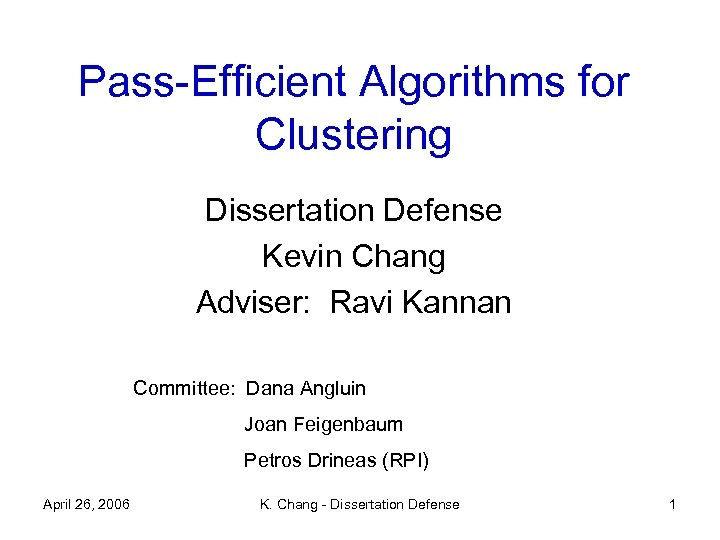Pass-Efficient Algorithms for Clustering Dissertation Defense Kevin Chang Adviser: Ravi Kannan Committee: Dana Angluin Joan Feigenbaum Petros Drineas (RPI) April 26, 2006 K. Chang - Dissertation Defense 1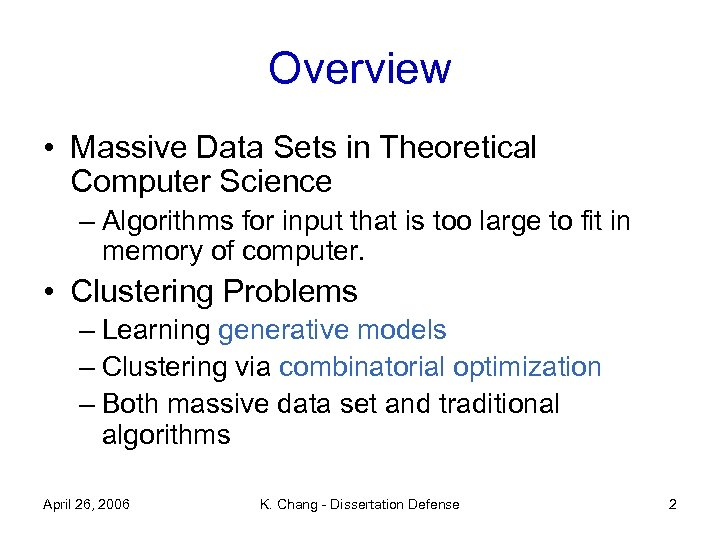Overview • Massive Data Sets in Theoretical Computer Science – Algorithms for input that is too large to fit in memory of computer. • Clustering Problems – Learning generative models – Clustering via combinatorial optimization – Both massive data set and traditional algorithms April 26, 2006 K. Chang - Dissertation Defense 2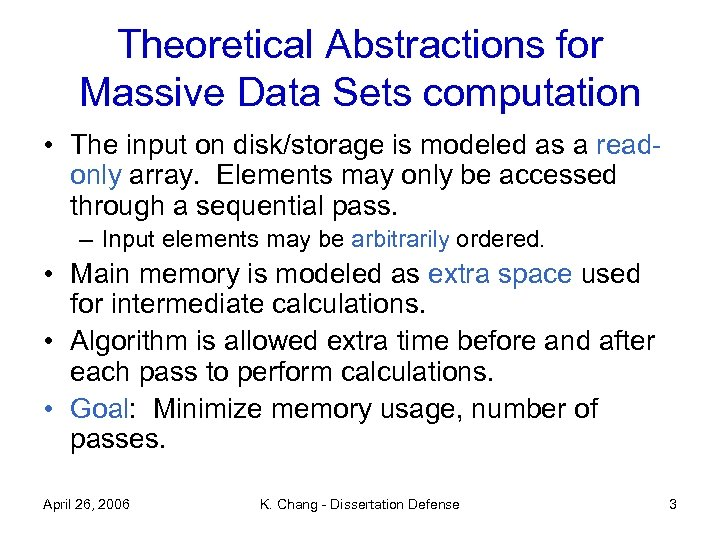Theoretical Abstractions for Massive Data Sets computation • The input on disk/storage is modeled as a readonly array. Elements may only be accessed through a sequential pass. – Input elements may be arbitrarily ordered. • Main memory is modeled as extra space used for intermediate calculations. • Algorithm is allowed extra time before and after each pass to perform calculations. • Goal: Minimize memory usage, number of passes. April 26, 2006 K. Chang - Dissertation Defense 3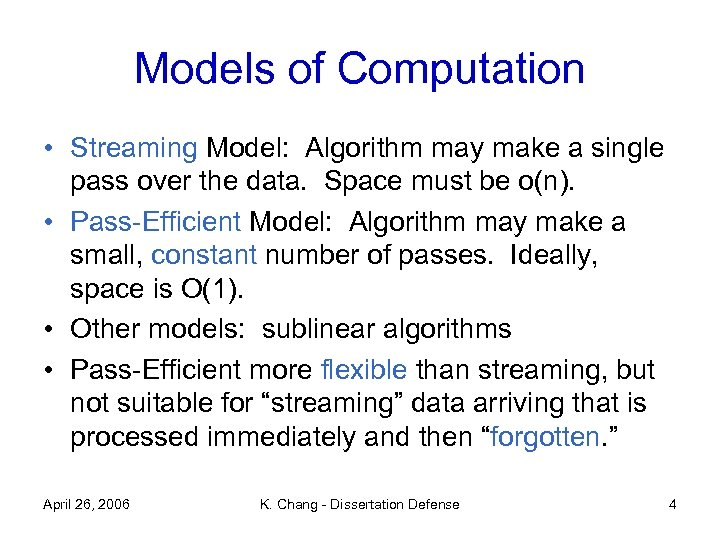Models of Computation • Streaming Model: Algorithm may make a single pass over the data. Space must be o(n). • Pass-Efficient Model: Algorithm may make a small, constant number of passes. Ideally, space is O(1). • Other models: sublinear algorithms • Pass-Efficient more flexible than streaming, but not suitable for “streaming” data arriving that is processed immediately and then “forgotten. ” April 26, 2006 K. Chang - Dissertation Defense 4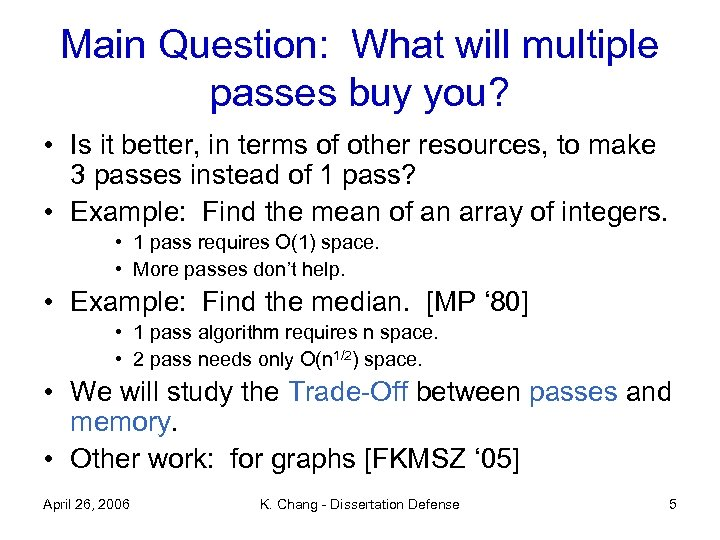Main Question: What will multiple passes buy you? • Is it better, in terms of other resources, to make 3 passes instead of 1 pass? • Example: Find the mean of an array of integers. • 1 pass requires O(1) space. • More passes don’t help. • Example: Find the median. [MP ‘ 80] • 1 pass algorithm requires n space. • 2 pass needs only O(n 1/2) space. • We will study the Trade-Off between passes and memory. • Other work: for graphs [FKMSZ ‘ 05] April 26, 2006 K. Chang - Dissertation Defense 5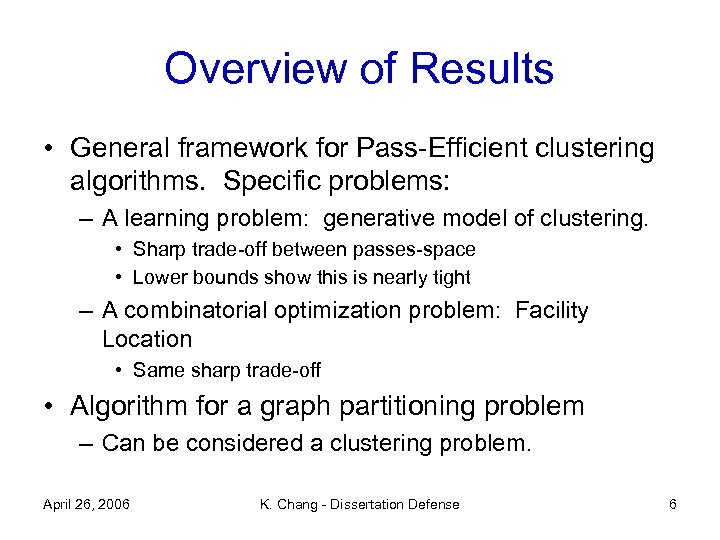Overview of Results • General framework for Pass-Efficient clustering algorithms. Specific problems: – A learning problem: generative model of clustering. • Sharp trade-off between passes-space • Lower bounds show this is nearly tight – A combinatorial optimization problem: Facility Location • Same sharp trade-off • Algorithm for a graph partitioning problem – Can be considered a clustering problem. April 26, 2006 K. Chang - Dissertation Defense 6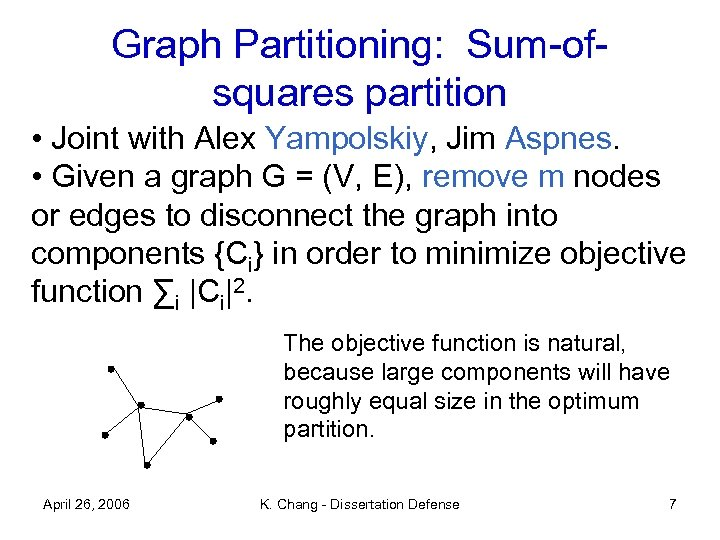Graph Partitioning: Sum-ofsquares partition • Joint with Alex Yampolskiy, Jim Aspnes. • Given a graph G = (V, E), remove m nodes or edges to disconnect the graph into components {Ci} in order to minimize objective function ∑i |Ci|2. The objective function is natural, because large components will have roughly equal size in the optimum partition. April 26, 2006 K. Chang - Dissertation Defense 7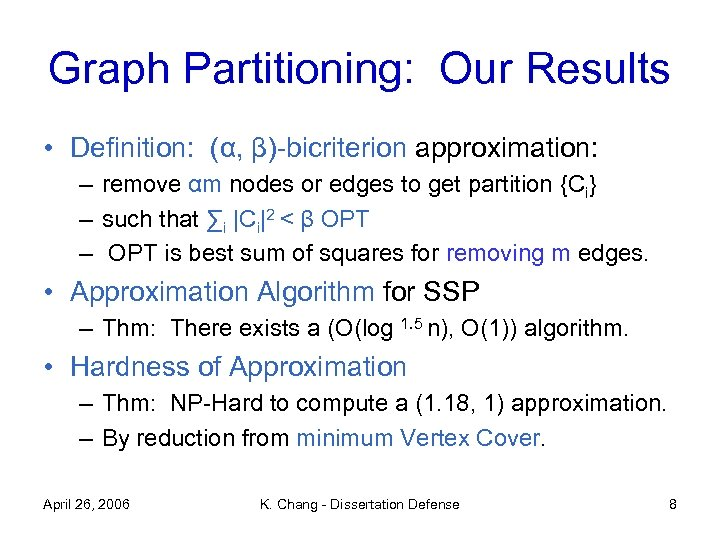Graph Partitioning: Our Results • Definition: (α, β)-bicriterion approximation: – remove αm nodes or edges to get partition {Ci} – such that ∑i |Ci|2 < β OPT – OPT is best sum of squares for removing m edges. • Approximation Algorithm for SSP – Thm: There exists a (O(log 1. 5 n), O(1)) algorithm. • Hardness of Approximation – Thm: NP-Hard to compute a (1. 18, 1) approximation. – By reduction from minimum Vertex Cover. April 26, 2006 K. Chang - Dissertation Defense 8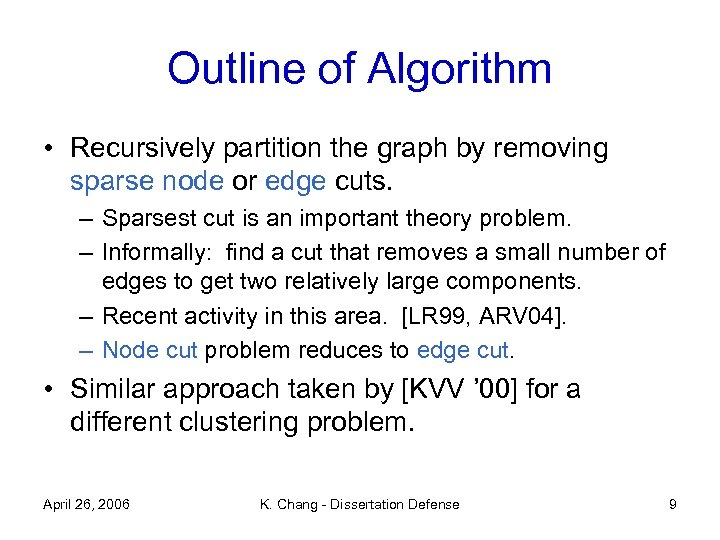Outline of Algorithm • Recursively partition the graph by removing sparse node or edge cuts. – Sparsest cut is an important theory problem. – Informally: find a cut that removes a small number of edges to get two relatively large components. – Recent activity in this area. [LR 99, ARV 04]. – Node cut problem reduces to edge cut. • Similar approach taken by [KVV ’ 00] for a different clustering problem. April 26, 2006 K. Chang - Dissertation Defense 9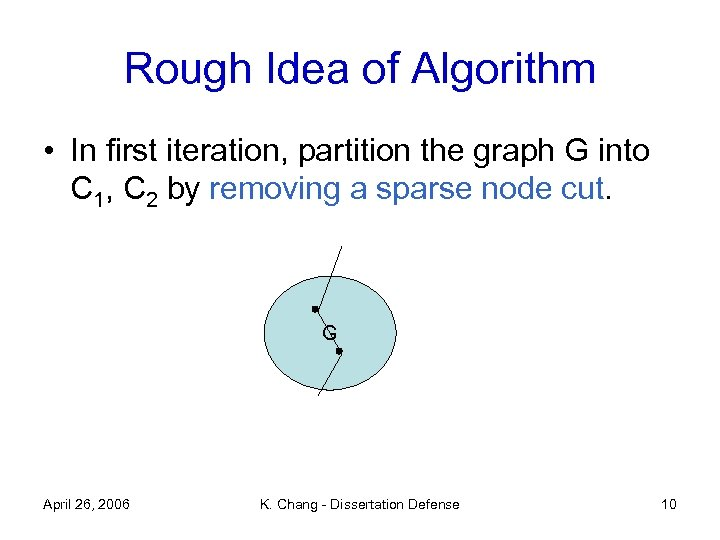Rough Idea of Algorithm • In first iteration, partition the graph G into C 1, C 2 by removing a sparse node cut. G April 26, 2006 K. Chang - Dissertation Defense 10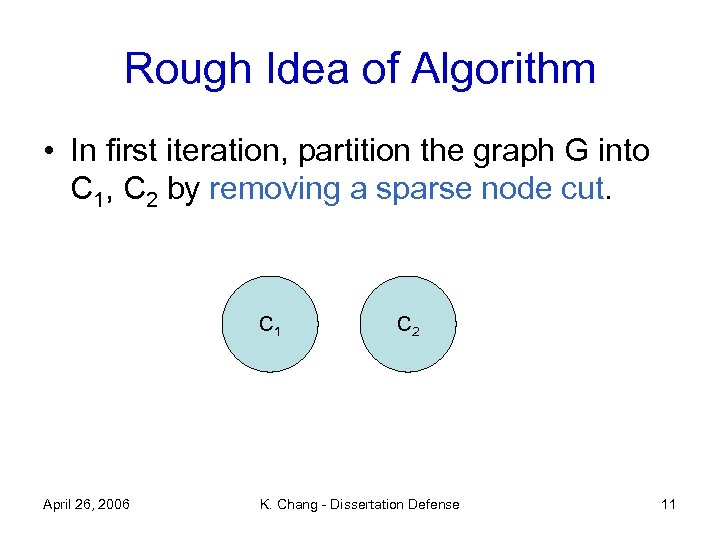Rough Idea of Algorithm • In first iteration, partition the graph G into C 1, C 2 by removing a sparse node cut. C 1 April 26, 2006 C 2 K. Chang - Dissertation Defense 11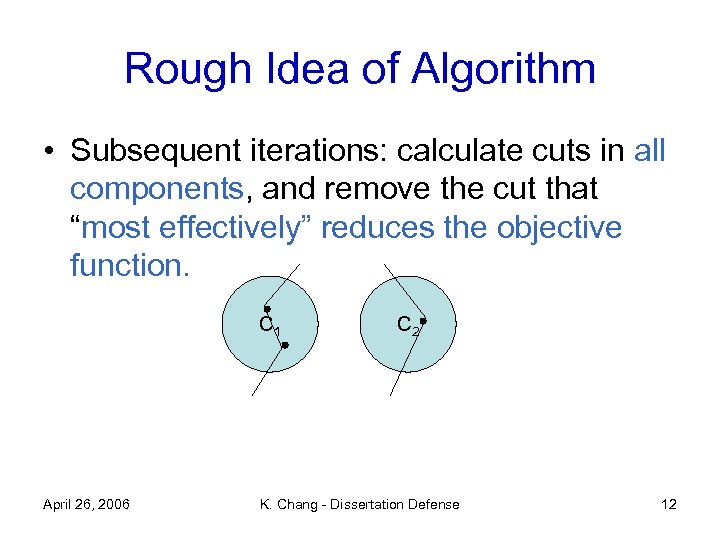Rough Idea of Algorithm • Subsequent iterations: calculate cuts in all components, and remove the cut that “most effectively” reduces the objective function. C 1 April 26, 2006 C 2 K. Chang - Dissertation Defense 12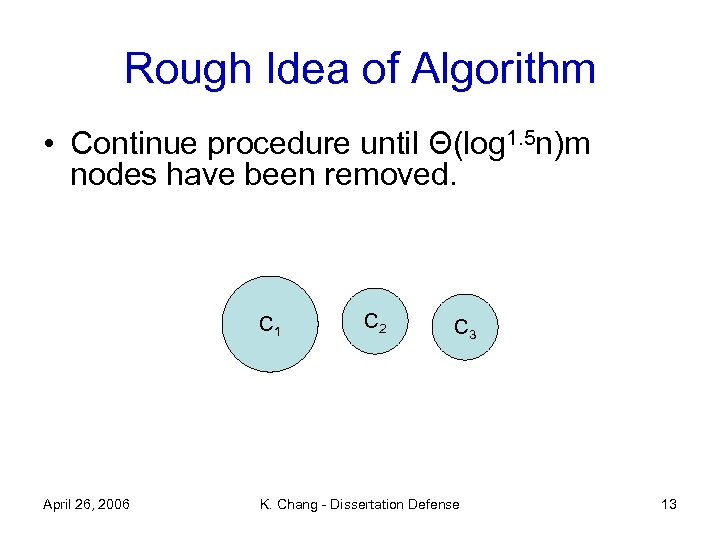Rough Idea of Algorithm • Continue procedure until Θ(log 1. 5 n)m nodes have been removed. C 1 April 26, 2006 C 2 C 3 K. Chang - Dissertation Defense 13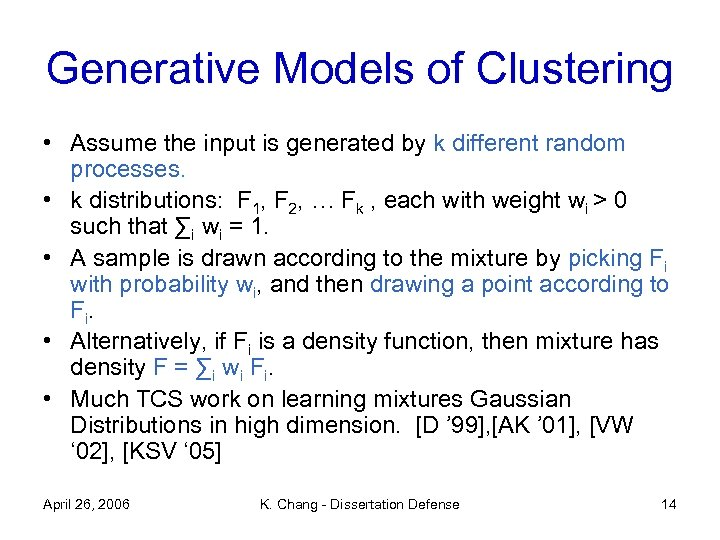Generative Models of Clustering • Assume the input is generated by k different random processes. • k distributions: F 1, F 2, … Fk , each with weight wi > 0 such that ∑i wi = 1. • A sample is drawn according to the mixture by picking Fi with probability wi, and then drawing a point according to F i. • Alternatively, if Fi is a density function, then mixture has density F = ∑i wi Fi. • Much TCS work on learning mixtures Gaussian Distributions in high dimension. [D ’ 99], [AK ’ 01], [VW ‘ 02], [KSV ‘ 05] April 26, 2006 K. Chang - Dissertation Defense 14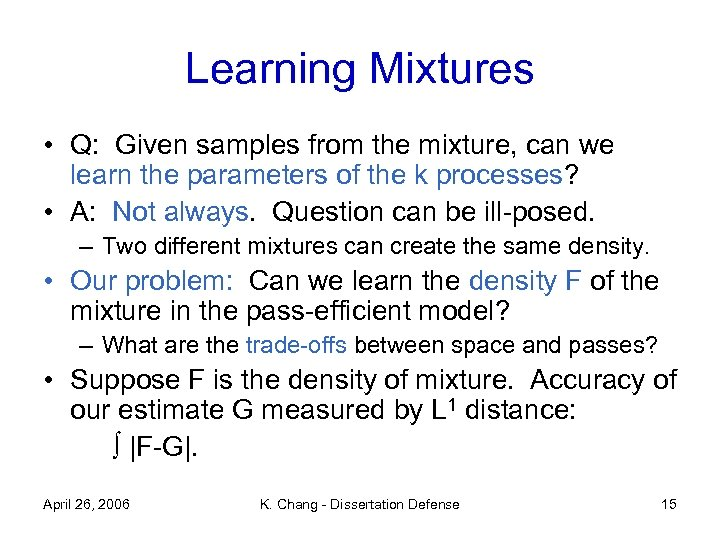Learning Mixtures • Q: Given samples from the mixture, can we learn the parameters of the k processes? • A: Not always. Question can be ill-posed. – Two different mixtures can create the same density. • Our problem: Can we learn the density F of the mixture in the pass-efficient model? – What are the trade-offs between space and passes? • Suppose F is the density of mixture. Accuracy of our estimate G measured by L 1 distance: ∫ |F-G|. April 26, 2006 K. Chang - Dissertation Defense 15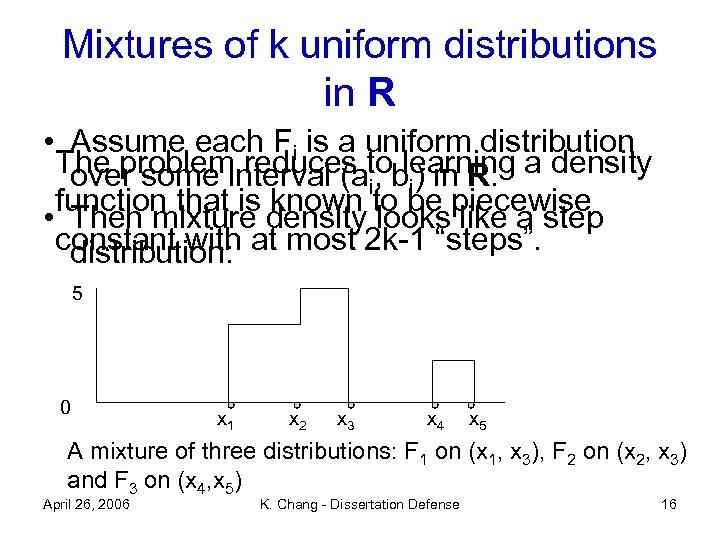Mixtures of k uniform distributions in R • Assume each Fi is a uniform distribution The probleminterval (atob ) in R. a density over some reduces i, learning i function that is known to be piecewise • Then mixture density looks like a step constant with at most 2 k-1 “steps”. distribution: 5 0 x 1 x 2 x 3 x 4 x 5 A mixture of three distributions: F 1 on (x 1, x 3), F 2 on (x 2, x 3) and F 3 on (x 4, x 5) April 26, 2006 K. Chang - Dissertation Defense 16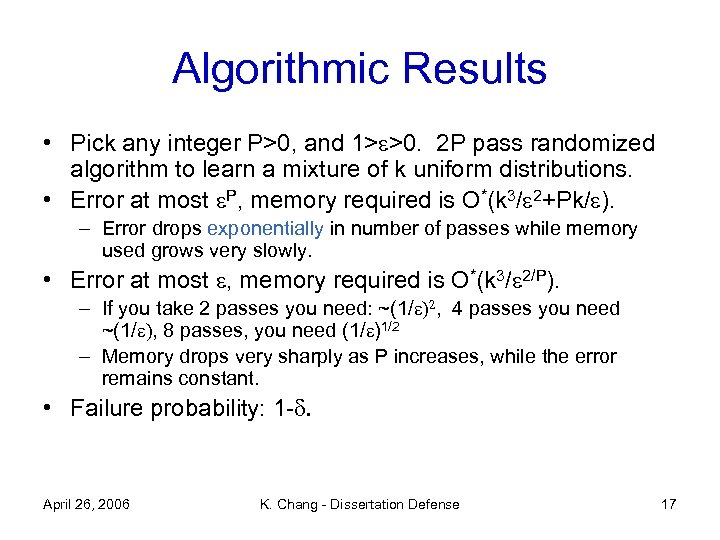Algorithmic Results • Pick any integer P>0, and 1> >0. 2 P pass randomized algorithm to learn a mixture of k uniform distributions. • Error at most P, memory required is O*(k 3/ 2+Pk/ ). – Error drops exponentially in number of passes while memory used grows very slowly. • Error at most , memory required is O*(k 3/ 2/P). – If you take 2 passes you need: ~(1/ )2, 4 passes you need ~(1/ ), 8 passes, you need (1/ )1/2 – Memory drops very sharply as P increases, while the error remains constant. • Failure probability: 1 -. April 26, 2006 K. Chang - Dissertation Defense 17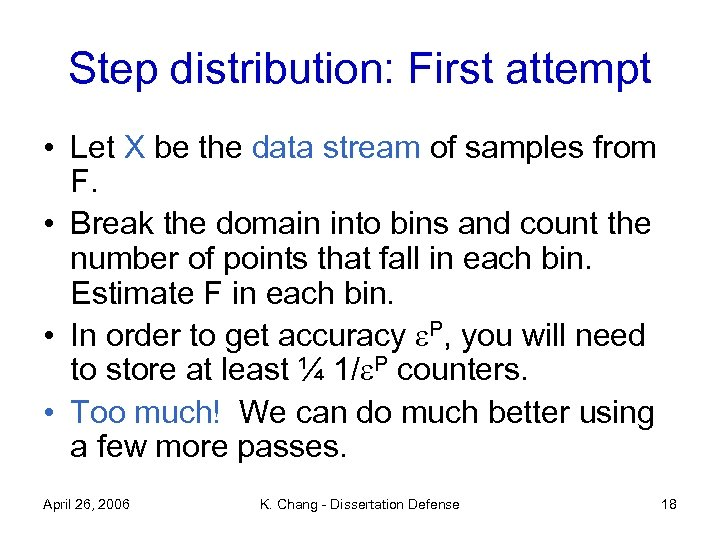Step distribution: First attempt • Let X be the data stream of samples from F. • Break the domain into bins and count the number of points that fall in each bin. Estimate F in each bin. • In order to get accuracy P, you will need to store at least ¼ 1/ P counters. • Too much! We can do much better using a few more passes. April 26, 2006 K. Chang - Dissertation Defense 18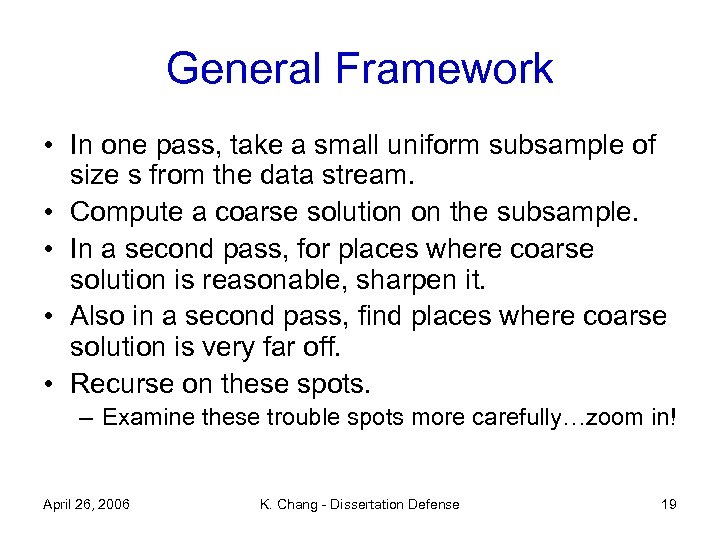General Framework • In one pass, take a small uniform subsample of size s from the data stream. • Compute a coarse solution on the subsample. • In a second pass, for places where coarse solution is reasonable, sharpen it. • Also in a second pass, find places where coarse solution is very far off. • Recurse on these spots. – Examine these trouble spots more carefully…zoom in! April 26, 2006 K. Chang - Dissertation Defense 19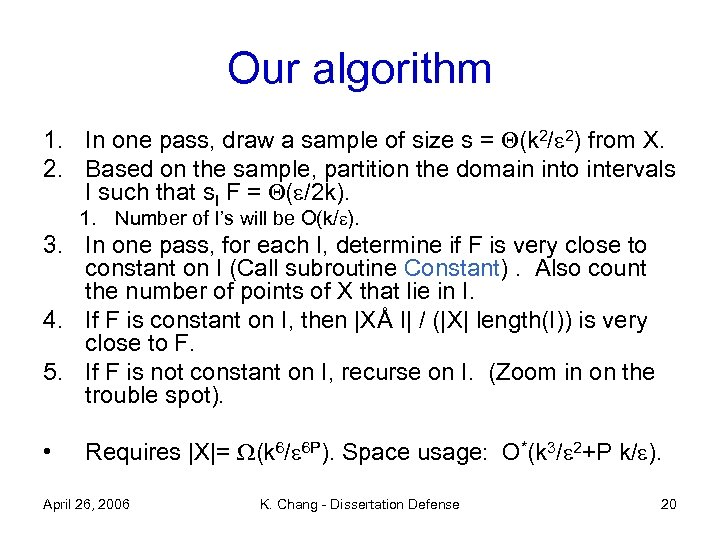Our algorithm 1. In one pass, draw a sample of size s = (k 2/ 2) from X. 2. Based on the sample, partition the domain into intervals I such that s. I F = ( /2 k). 1. Number of I’s will be O(k/ ). 3. In one pass, for each I, determine if F is very close to constant on I (Call subroutine Constant). Also count the number of points of X that lie in I. 4. If F is constant on I, then |XÅ I| / (|X| length(I)) is very close to F. 5. If F is not constant on I, recurse on I. (Zoom in on the trouble spot). • Requires |X|= (k 6/ 6 P). Space usage: O*(k 3/ 2+P k/ ). April 26, 2006 K. Chang - Dissertation Defense 20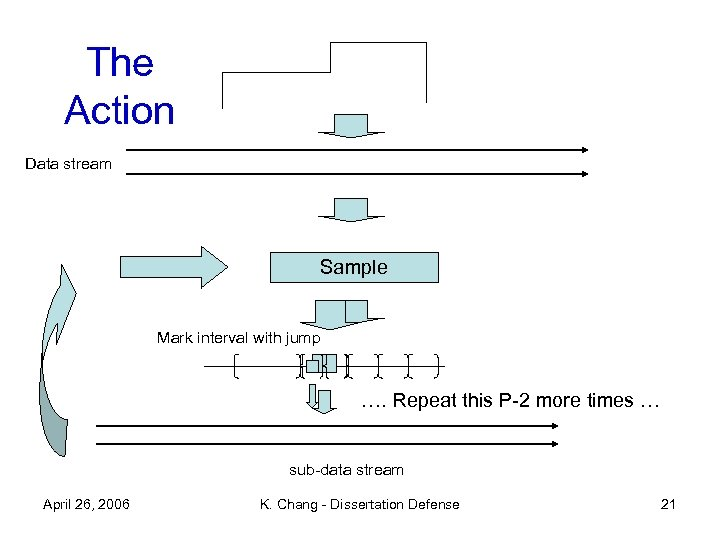The Action Data stream Sample Mark interval with jump …. Repeat this P-2 more times … sub-data stream April 26, 2006 K. Chang - Dissertation Defense 21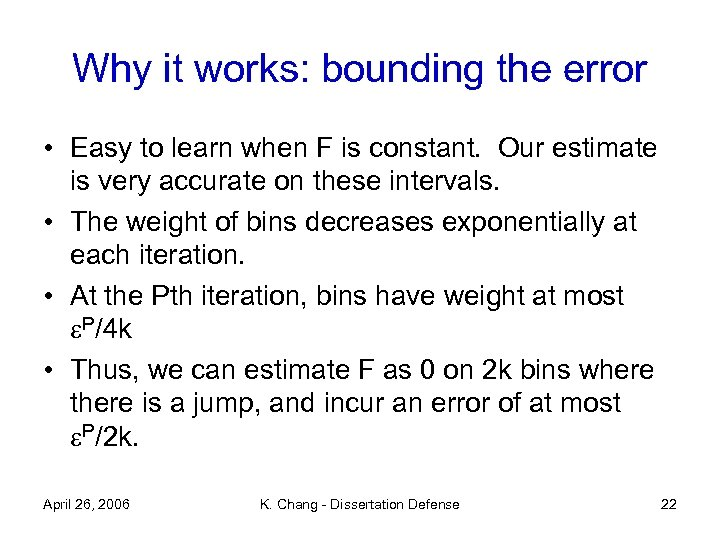Why it works: bounding the error • Easy to learn when F is constant. Our estimate is very accurate on these intervals. • The weight of bins decreases exponentially at each iteration. • At the Pth iteration, bins have weight at most P/4 k • Thus, we can estimate F as 0 on 2 k bins where there is a jump, and incur an error of at most P/2 k. April 26, 2006 K. Chang - Dissertation Defense 22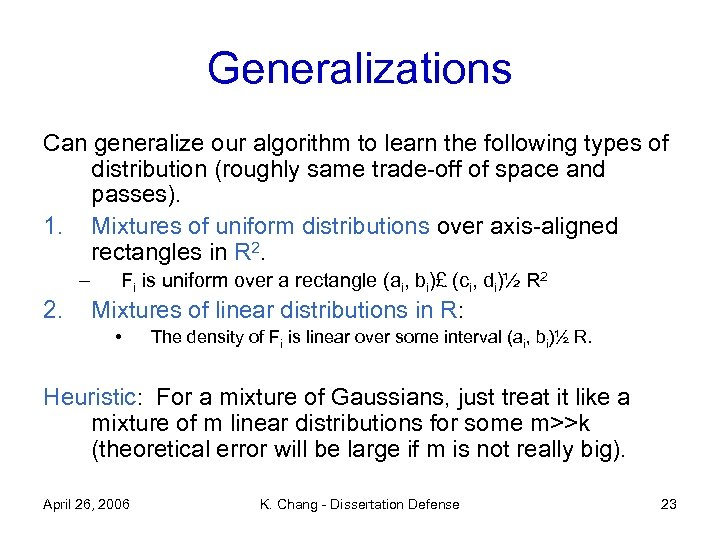Generalizations Can generalize our algorithm to learn the following types of distribution (roughly same trade-off of space and passes). 1. Mixtures of uniform distributions over axis-aligned rectangles in R 2. – 2. Fi is uniform over a rectangle (ai, bi)£ (ci, di)½ R 2 Mixtures of linear distributions in R: • The density of Fi is linear over some interval (ai, bi)½ R. Heuristic: For a mixture of Gaussians, just treat it like a mixture of m linear distributions for some m>>k (theoretical error will be large if m is not really big). April 26, 2006 K. Chang - Dissertation Defense 23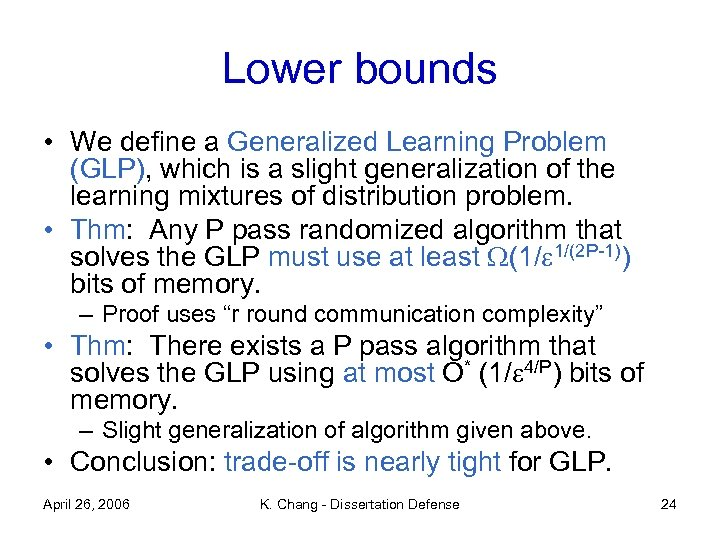Lower bounds • We define a Generalized Learning Problem (GLP), which is a slight generalization of the learning mixtures of distribution problem. • Thm: Any P pass randomized algorithm that solves the GLP must use at least (1/ 1/(2 P-1)) bits of memory. – Proof uses “r round communication complexity” • Thm: There exists a P pass algorithm that solves the GLP using at most O* (1/ 4/P) bits of memory. – Slight generalization of algorithm given above. • Conclusion: trade-off is nearly tight for GLP. April 26, 2006 K. Chang - Dissertation Defense 24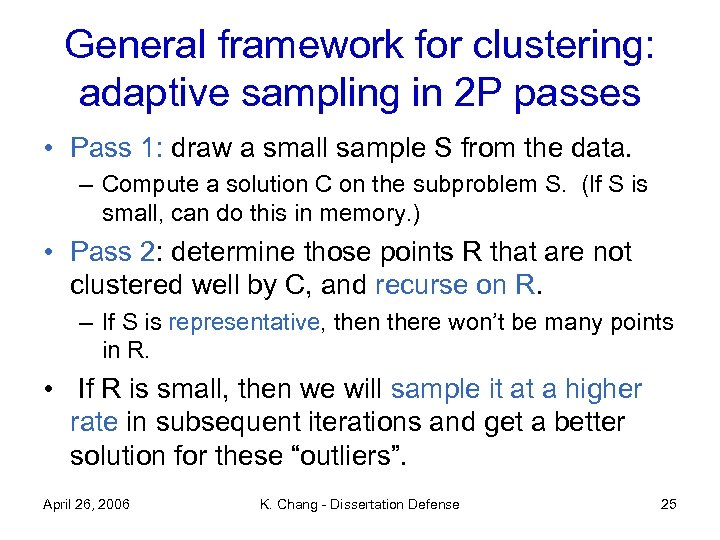General framework for clustering: adaptive sampling in 2 P passes • Pass 1: draw a small sample S from the data. – Compute a solution C on the subproblem S. (If S is small, can do this in memory. ) • Pass 2: determine those points R that are not clustered well by C, and recurse on R. – If S is representative, then there won’t be many points in R. • If R is small, then we will sample it at a higher rate in subsequent iterations and get a better solution for these “outliers”. April 26, 2006 K. Chang - Dissertation Defense 25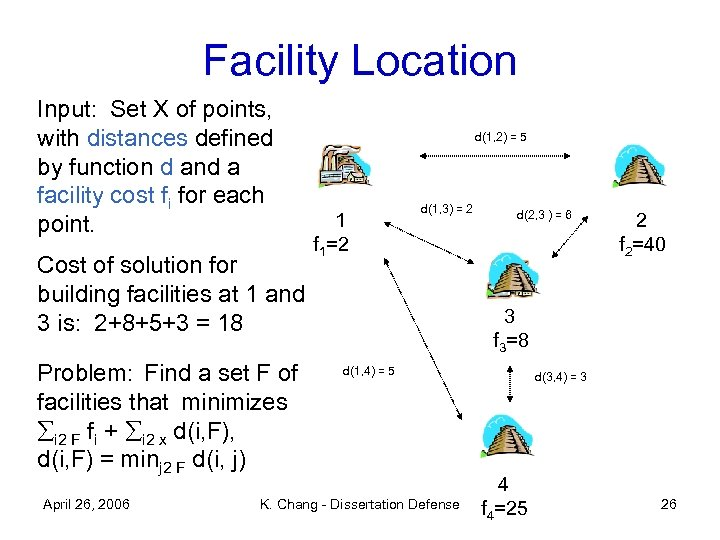Facility Location Input: Set X of points, with distances defined by function d and a facility cost fi for each point. Cost of solution for building facilities at 1 and 3 is: 2+8+5+3 = 18 Problem: Find a set F of facilities that minimizes i 2 F fi + i 2 x d(i, F), d(i, F) = minj 2 F d(i, j) April 26, 2006 d(1, 2) = 5 1 f 1=2 d(1, 3) = 2 d(2, 3 ) = 6 2 f 2=40 3 f 3=8 d(1, 4) = 5 K. Chang - Dissertation Defense d(3, 4) = 3 4 f 4=25 26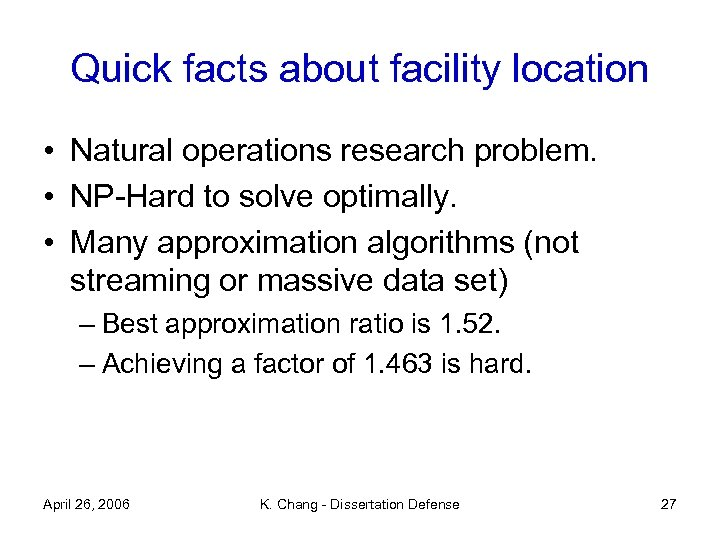Quick facts about facility location • Natural operations research problem. • NP-Hard to solve optimally. • Many approximation algorithms (not streaming or massive data set) – Best approximation ratio is 1. 52. – Achieving a factor of 1. 463 is hard. April 26, 2006 K. Chang - Dissertation Defense 27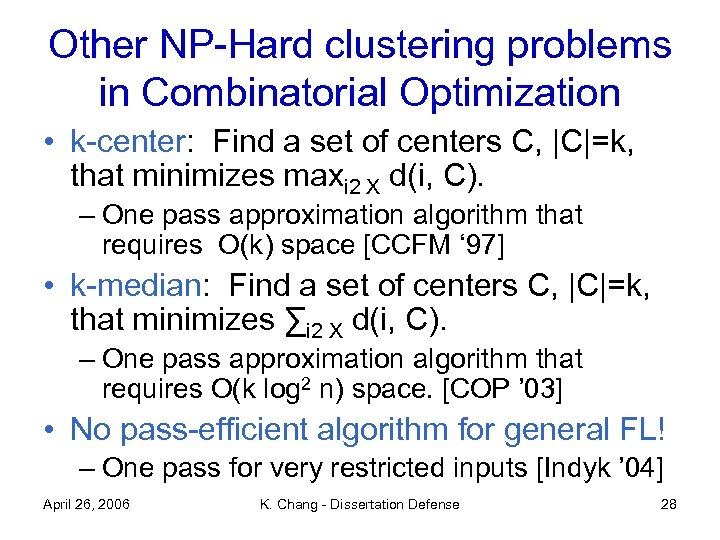Other NP-Hard clustering problems in Combinatorial Optimization • k-center: Find a set of centers C, |C|=k, that minimizes maxi 2 X d(i, C). – One pass approximation algorithm that requires O(k) space [CCFM ‘ 97] • k-median: Find a set of centers C, |C|=k, that minimizes ∑i 2 X d(i, C). – One pass approximation algorithm that requires O(k log 2 n) space. [COP ’ 03] • No pass-efficient algorithm for general FL! – One pass for very restricted inputs [Indyk ’ 04] April 26, 2006 K. Chang - Dissertation Defense 28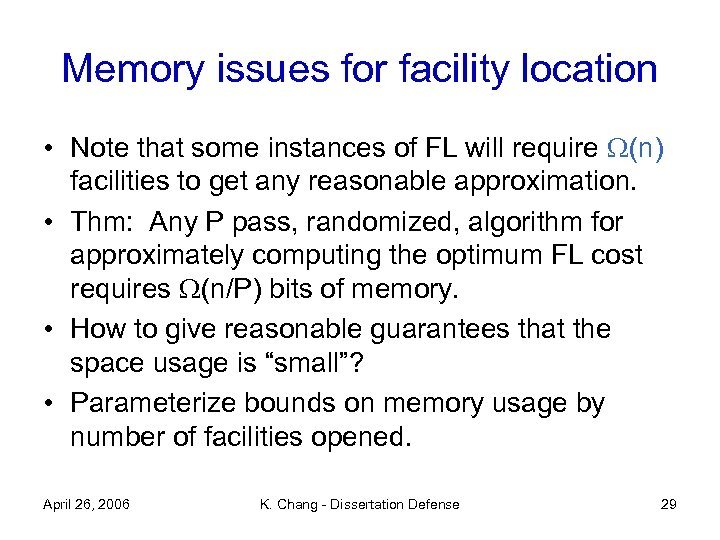Memory issues for facility location • Note that some instances of FL will require (n) facilities to get any reasonable approximation. • Thm: Any P pass, randomized, algorithm for approximately computing the optimum FL cost requires (n/P) bits of memory. • How to give reasonable guarantees that the space usage is “small”? • Parameterize bounds on memory usage by number of facilities opened. April 26, 2006 K. Chang - Dissertation Defense 29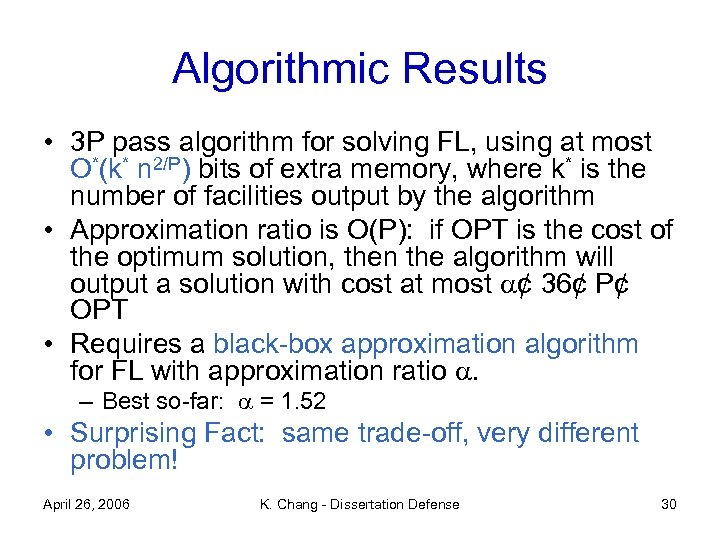Algorithmic Results • 3 P pass algorithm for solving FL, using at most O*(k* n 2/P) bits of extra memory, where k* is the number of facilities output by the algorithm • Approximation ratio is O(P): if OPT is the cost of the optimum solution, then the algorithm will output a solution with cost at most ¢ 36¢ P¢ OPT • Requires a black-box approximation algorithm for FL with approximation ratio . – Best so-far: = 1. 52 • Surprising Fact: same trade-off, very different problem! April 26, 2006 K. Chang - Dissertation Defense 30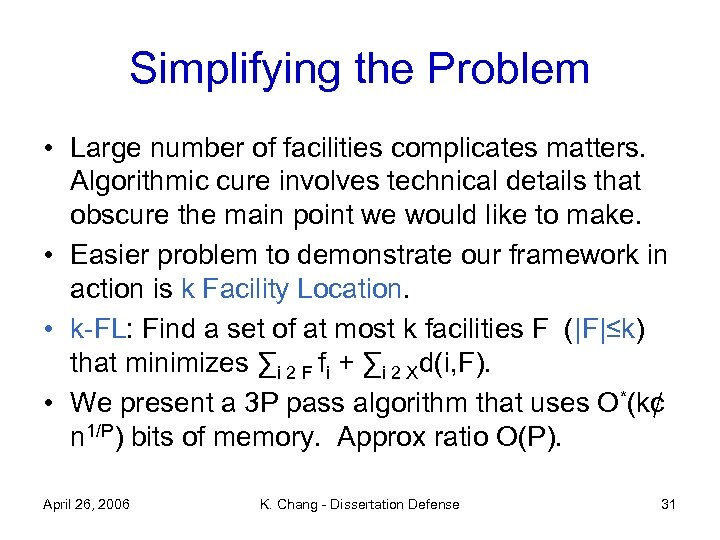Simplifying the Problem • Large number of facilities complicates matters. Algorithmic cure involves technical details that obscure the main point we would like to make. • Easier problem to demonstrate our framework in action is k Facility Location. • k-FL: Find a set of at most k facilities F (|F|≤k) that minimizes ∑i 2 F fi + ∑i 2 Xd(i, F). • We present a 3 P pass algorithm that uses O*(k¢ n 1/P) bits of memory. Approx ratio O(P). April 26, 2006 K. Chang - Dissertation Defense 31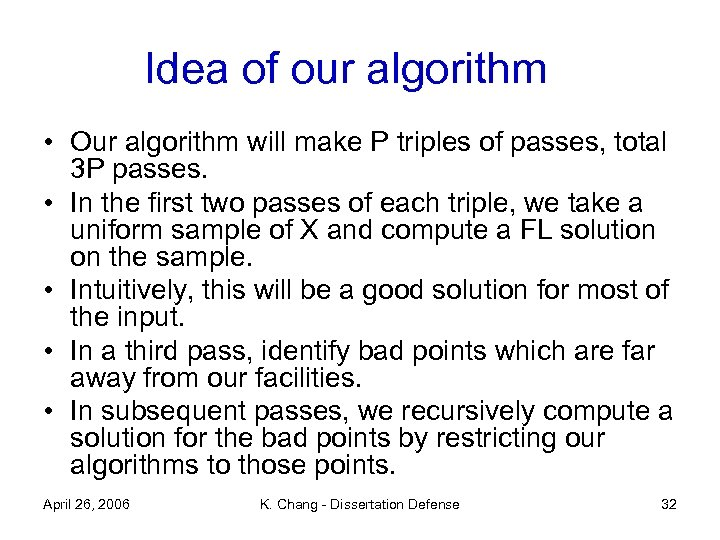Idea of our algorithm • Our algorithm will make P triples of passes, total 3 P passes. • In the first two passes of each triple, we take a uniform sample of X and compute a FL solution on the sample. • Intuitively, this will be a good solution for most of the input. • In a third pass, identify bad points which are far away from our facilities. • In subsequent passes, we recursively compute a solution for the bad points by restricting our algorithms to those points. April 26, 2006 K. Chang - Dissertation Defense 32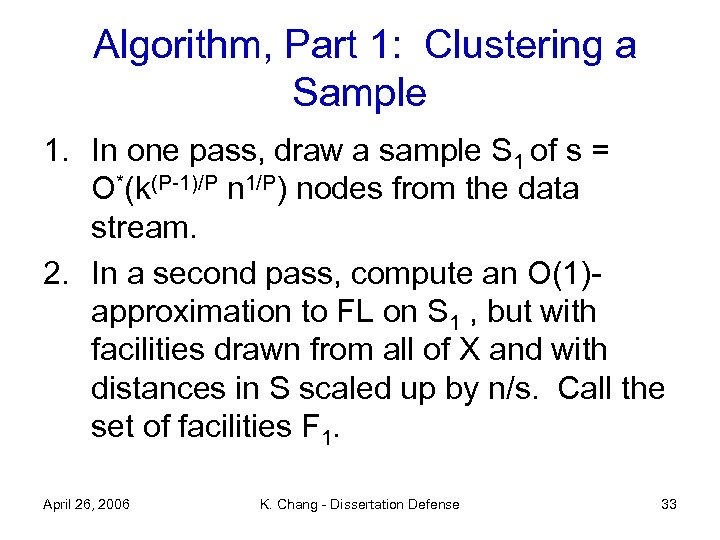Algorithm, Part 1: Clustering a Sample 1. In one pass, draw a sample S 1 of s = O*(k(P-1)/P n 1/P) nodes from the data stream. 2. In a second pass, compute an O(1)approximation to FL on S 1 , but with facilities drawn from all of X and with distances in S scaled up by n/s. Call the set of facilities F 1. April 26, 2006 K. Chang - Dissertation Defense 33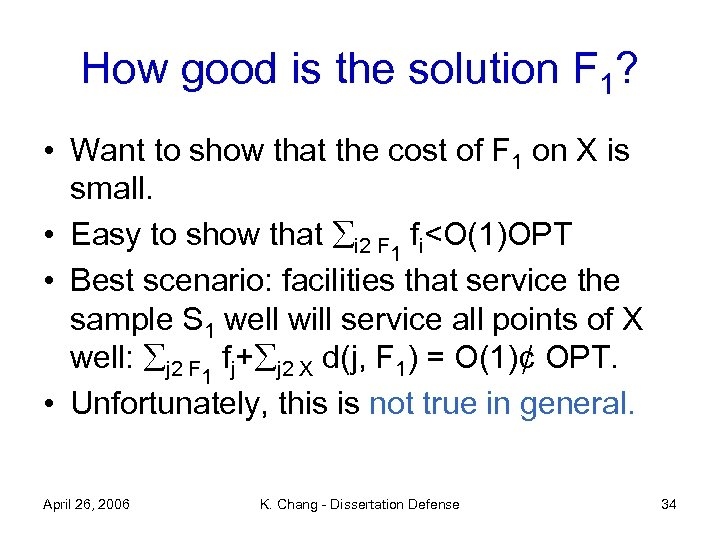How good is the solution F 1? • Want to show that the cost of F 1 on X is small. • Easy to show that i 2 F 1 fi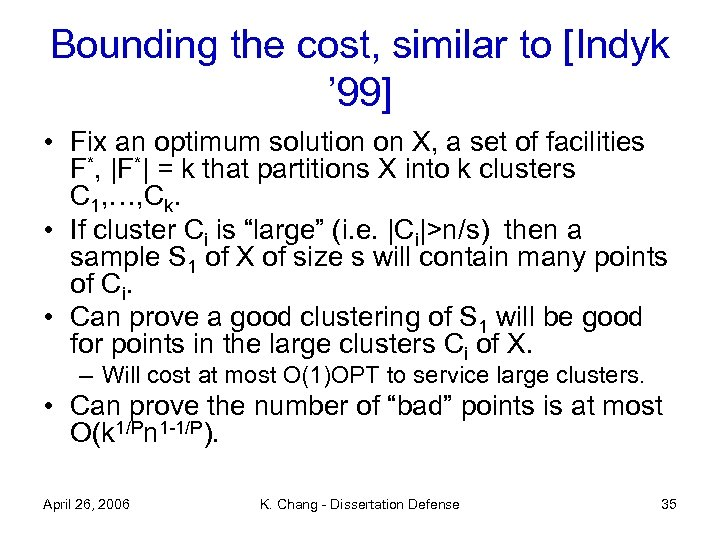Bounding the cost, similar to [Indyk ’ 99] • Fix an optimum solution on X, a set of facilities F*, |F*| = k that partitions X into k clusters C 1, …, Ck. • If cluster Ci is “large” (i. e. |Ci|>n/s) then a sample S 1 of X of size s will contain many points of Ci. • Can prove a good clustering of S 1 will be good for points in the large clusters Ci of X. – Will cost at most O(1)OPT to service large clusters. • Can prove the number of “bad” points is at most O(k 1/Pn 1 -1/P). April 26, 2006 K. Chang - Dissertation Defense 35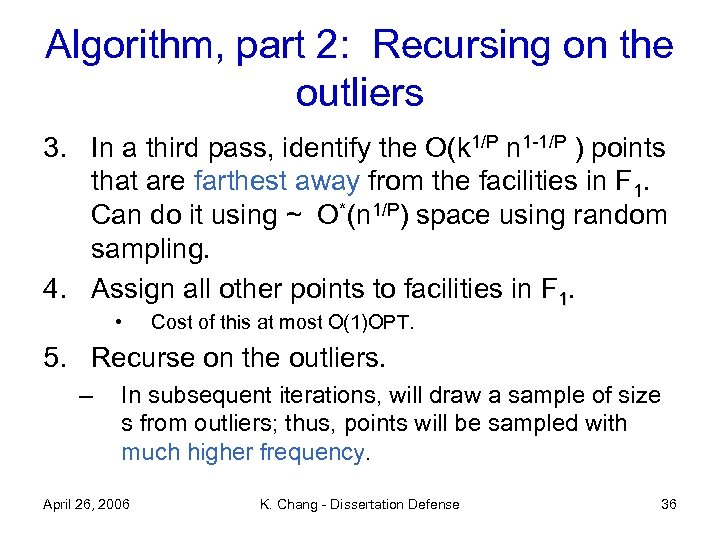Algorithm, part 2: Recursing on the outliers 3. In a third pass, identify the O(k 1/P n 1 -1/P ) points that are farthest away from the facilities in F 1. Can do it using ~ O*(n 1/P) space using random sampling. 4. Assign all other points to facilities in F 1. • Cost of this at most O(1)OPT. 5. Recurse on the outliers. – In subsequent iterations, will draw a sample of size s from outliers; thus, points will be sampled with much higher frequency. April 26, 2006 K. Chang - Dissertation Defense 36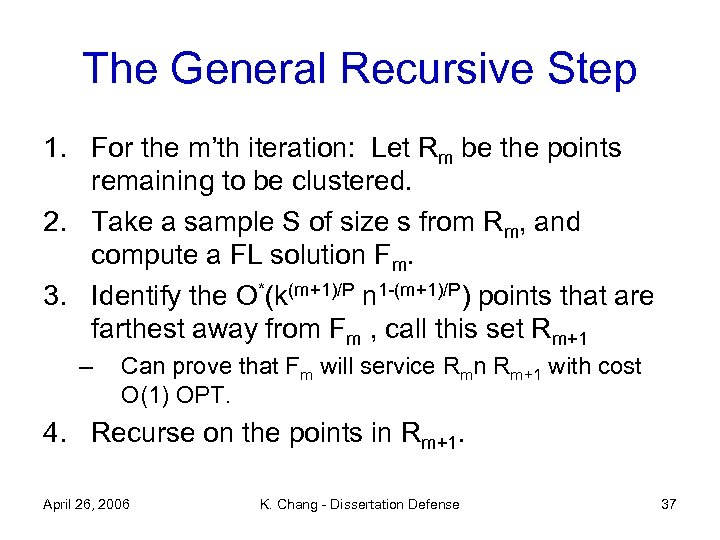The General Recursive Step 1. For the m’th iteration: Let Rm be the points remaining to be clustered. 2. Take a sample S of size s from Rm, and compute a FL solution Fm. 3. Identify the O*(k(m+1)/P n 1 -(m+1)/P) points that are farthest away from Fm , call this set Rm+1 – Can prove that Fm will service Rmn Rm+1 with cost O(1) OPT. 4. Recurse on the points in Rm+1. April 26, 2006 K. Chang - Dissertation Defense 37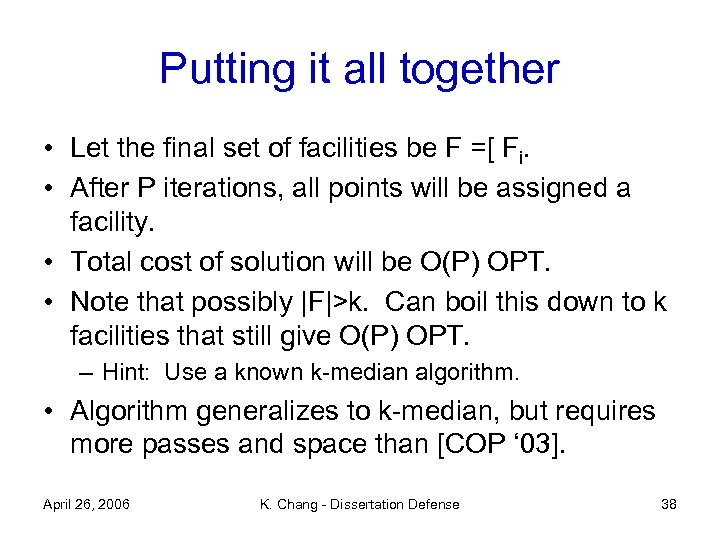Putting it all together • Let the final set of facilities be F =[ Fi. • After P iterations, all points will be assigned a facility. • Total cost of solution will be O(P) OPT. • Note that possibly |F|>k. Can boil this down to k facilities that still give O(P) OPT. – Hint: Use a known k-median algorithm. • Algorithm generalizes to k-median, but requires more passes and space than [COP ‘ 03]. April 26, 2006 K. Chang - Dissertation Defense 38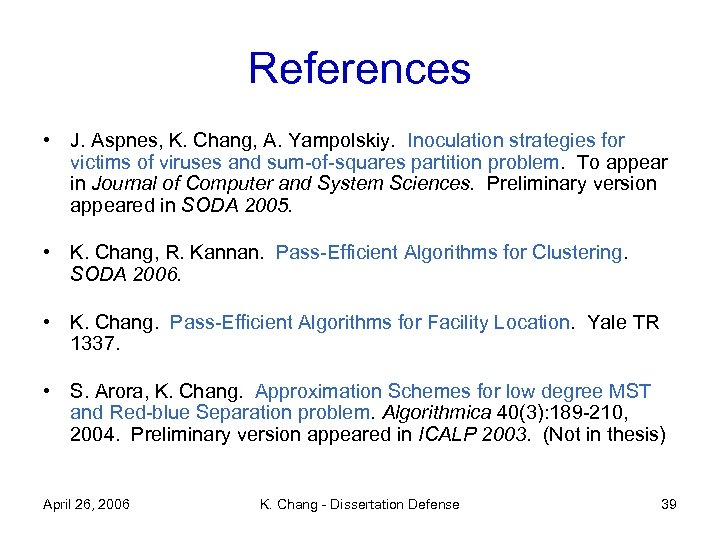References • J. Aspnes, K. Chang, A. Yampolskiy. Inoculation strategies for victims of viruses and sum-of-squares partition problem. To appear in Journal of Computer and System Sciences. Preliminary version appeared in SODA 2005. • K. Chang, R. Kannan. Pass-Efficient Algorithms for Clustering. SODA 2006. • K. Chang. Pass-Efficient Algorithms for Facility Location. Yale TR 1337. • S. Arora, K. Chang. Approximation Schemes for low degree MST and Red-blue Separation problem. Algorithmica 40(3): 189 -210, 2004. Preliminary version appeared in ICALP 2003. (Not in thesis) April 26, 2006 K. Chang - Dissertation Defense 39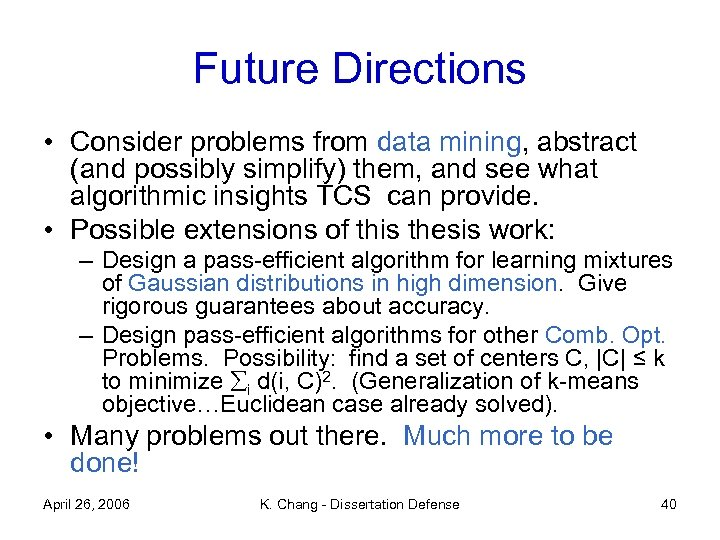Future Directions • Consider problems from data mining, abstract (and possibly simplify) them, and see what algorithmic insights TCS can provide. • Possible extensions of this thesis work: – Design a pass-efficient algorithm for learning mixtures of Gaussian distributions in high dimension. Give rigorous guarantees about accuracy. – Design pass-efficient algorithms for other Comb. Opt. Problems. Possibility: find a set of centers C, |C| ≤ k to minimize i d(i, C)2. (Generalization of k-means objective…Euclidean case already solved). • Many problems out there. Much more to be done! April 26, 2006 K. Chang - Dissertation Defense 40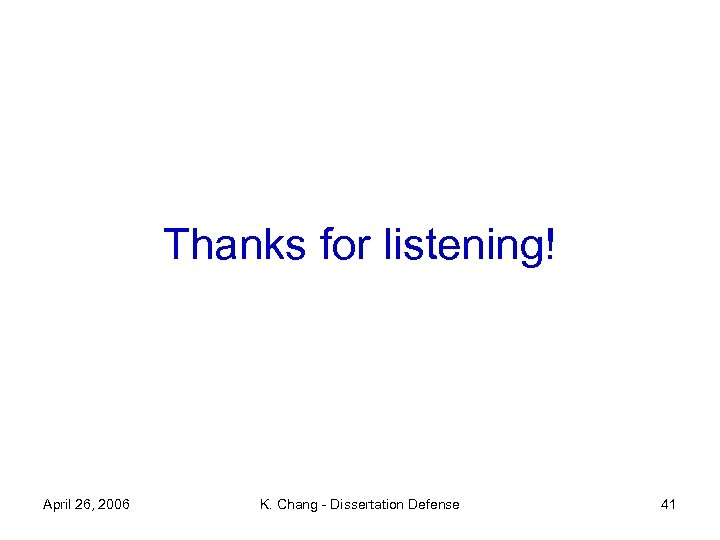Thanks for listening! April 26, 2006 K. Chang - Dissertation Defense 41